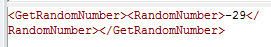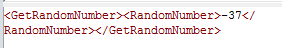Top Top
 Library > EASYProcess Reference Manual > Processes > Services > GeneralUtilityWorkshop > GetRandomNumber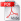GetRandomNumberGetRandomNumber can be used to generate an unpredictable/random number from a given range. This service could be helpful when a random value is needed, such as a user’s initial password on user creation.Once the service is dragged onto the Process Canvas, it is auto-filled with default values: minimum (1000000) and maximum (9999999).The endpoints (Min and Max Values) of the range chosen are possible selections for the resulting Random Number.
 GetRandomNumber Use Examples
 GetRandomNumber Exceptions
 Varying Min and Max Digit Lengths
 EndPoints Included
 Range of Negative Numbers
 Workshop: GeneralUtilityWorkshop Service: GetRandomNumber
 Inputs
 MinValue This is the value that you will assign as the minimum value. It will be the minimum value that the generator can generate.
 MaxValue This is the value that you will assign as the maximum value. It will be the maximum value that the generator can generate.
 Outputs
 RandomNumber This is the value that the service gives as the random number from the selected range of numbers.GetRandomNumber Use Examples

# Basic Use Example

In the following example we have a button process which uses the GetRandomNumber service and displays it on a pop-up box on a web page.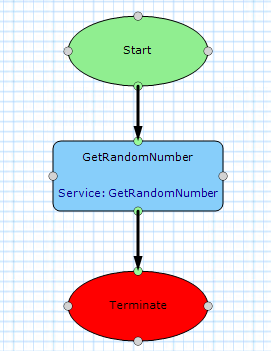Here is the service configuration. Notice the inputs have not been changed from the default values. This means the service was added to the Process Canvas and run without further configuration.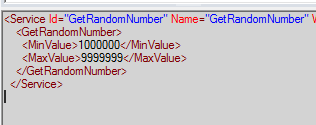When the service runs, the RandomNumber is returned in the output. Here are the service output logs.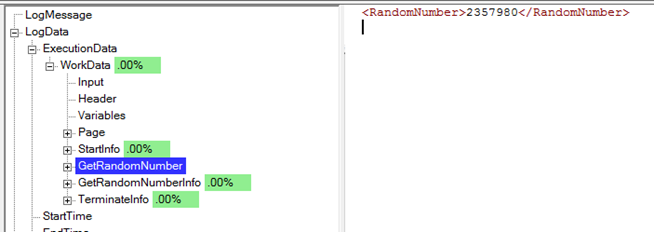In this example, when creating a new user, a random password is generated and sent to the new user’s email address. This is a temporary password that will only be used for the first login because the user will be prompted to change it on the first successful log in.

Here is the process canvas for this feature. First a random number is generated and then a portion of this large number its taken to be the password.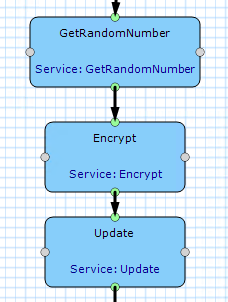Here are the service configurations. It does not use the default inputs for the GetRandomNumber services, because the number generated from the default values is only 7 digits long. This particular application example has a password complexity rule of 8 characters minimum, so the RandomNumber needs to be longer than this. The Min and Max values chosen are very large so the resulting number will also be very large and always over 8 characters. The Encrypt then takes a substring of the random number to be the user’s password. The substring is always the same length so that all user’s passwords are the same length for consistency.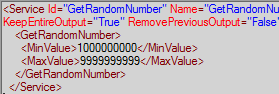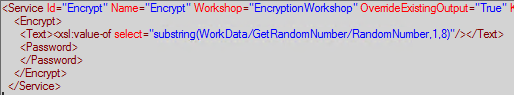Here is the service output. It is 9 characters long, so the first 8 characters will be taken as the user’s password.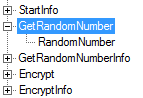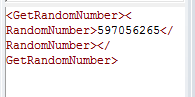GetRandomNumber ExceptionsThe GetRandomNumber service expects the MinValue to be less than the MaxValue. If this is not true, an exception will be thrown. See the Exception Message below: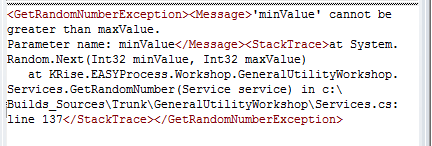Varying Min and Max Digit Lengths

In both examples so far, the MinValue and MaxValue have been chosen so that the resulting random number is always the same number of digits. However, if the MinValue and MaxValue vary in number of digits, so can the results.

In this example, we have chosen a wide range: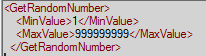The service has been run multiple times to demonstrate the possible outputs and their varying lengths.8 Digits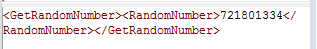9 Digits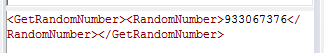9 Digits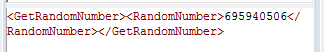9 Digits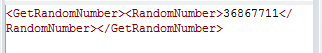8 Digits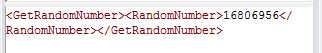8 Digits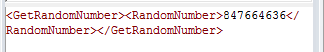9 Digits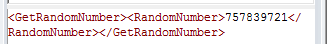9 Digits

Notice that even though all numbers between 1 and just short of a billion are possible, many 9 digits numbers are appearing and only a few 8 digit. This is because of the range chosen. Here is the count of how many numbers exist with each number of digits:

 1 Digit 9 numbers (1 - 9) .0000009% 2 Digit 90 numbers (10 - 99) .000009% 3 Digit 900 numbers (100 - 999) .00009% 4 Digit 9,000 numbers (1,000 - 9,999) .0009% 5 Digit 90,000 numbers (10,000 - 99,999) .009% 6 Digit 900,000 numbers (100,000 - 999,999) .09% 7 Digit 9,000,000 numbers (1,000,000 - 9,999,999) .9% 8 Digit 90,000,000 numbers (10,000,000 - 99,999,999) 9% 9 Digit 900,000,000 numbers (100,000,000 - 999,999,999) 90%

So while it is possible to get a 1 digit number when generating a random number in the range from 1 to 999,999,999 is possible, it is unlikely because there is only a 9/999999999 (.0000009%) chance it will occur.End Points IncludedThis example will show a tiny range to demonstrate that the end points are included in the selection range of the Random Number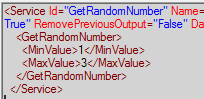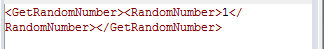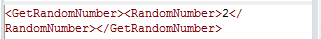Range of Negative NumbersGetRandomNumber can also take negative numbers and input and pick a negative number as the RandomNumber.Service Configuration: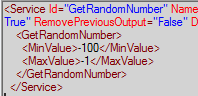Output: